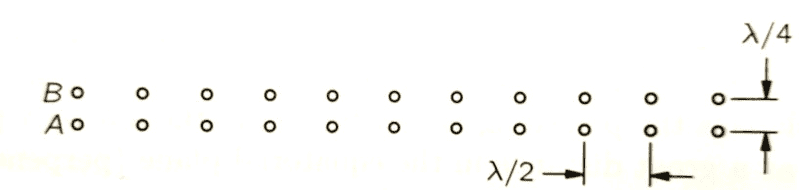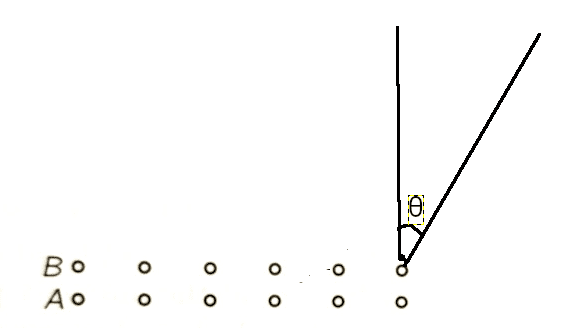# Calculating Radiation Pattern for a grid of two rows of dipole antennas

Homework Statement:
A double line of N equally spaced oscillating dipoles is situated as shown in figure (below). All dipoles in row A are driven in the same phase, and all those in row B lag 90° in phase behind those of row A. Sketch the radiation pattern I(θ) in the equatorial plane at a great distance from the array
Relevant Equations:
Based on Interference chapter in feynman lectures: https://www.feynmanlectures.caltech.edu/I_29.htmlAlthough problem says sketch, what I really want is the formulae by θ for the radiation. After trying this for a while I cheated and looked at the solution. I still can't figure out the steps on how to get to the solution. the answer is:
$$I(\theta) = 2I_0 [ 1 + cos(\frac{\pi}{2}(1-cos\theta)) ] \frac{sin^2(\frac{N\pi}{2}sin\theta)}{sin^2(\frac{\pi}{2}sin\theta)}$$

What I tried:

I'll assume that the intensity from a single antenna at a great distance is ##I_0## and I need to find what is the combined intensity after accounting for interference. To start with let's find out the phase shift for each antenna with respect to the top-right most antenna in row B as 0 phase shift. Ignoring the phase shift from distance, Every antenna in row A is ##\pi/2## ahead of row B.

Let us number the antenna in both rows starting with the right end as k=0 , k = 1,... k=N-1.

In row B, after doing the geometry the phase-shift per antenna in terms of distance is : ##k \frac{\lambda}{2} \sin\theta##. (Separated from top right antenna by a horizontal distance of ##k\frac{\lambda}{2}## & and target point is at an angle θ). So the total phase shift in row A is ##k\pi sin \theta##.

In row A, we need to do a slightly more complicated geometry, where the antenna is ##\frac{\lambda}{4}## vertically below & ##k\frac{\lambda}{2}## horizontally to the left. The distance between the two antenna is ##\frac{\lambda}{4}\sqrt{4k^2+1}##. The angle to the target point with respect to the line between the two antenna is ##tan^{-1}(\frac{1}{2k}) + \theta - \pi/2##. So the phase-shift in terms of distance is : ##\frac{\lambda}{4}\sqrt{4k^2+1} cos(tan^{-1}(\frac{1}{2k}) + \theta - \pi/2)## . Simplifying & in terms of phase shift in radians & adding the phase shift difference it is driven at, total phase shift: ##\frac{\pi}{2}(\sqrt{4k^2+1} sin(tan^{-1}(\frac{1}{2k}) + \theta)-1)##

So using complex numbers the combined Electric field would be represented by:

$$\hat{E} = \sum_{k=0}^{N-1} e^{i k\pi sin \theta} + \sum_{k=0}^{N-1} e^{i \frac{\pi}{2}(\sqrt{4k^2+1} sin(tan^{-1}(\frac{1}{2k}) + \theta)-1)}$$

The intensity should be the magnitude of ##\hat{E}## squared, which we can get by multiplying it by it's complex conjugate. But at this point the math become so complicated, that I couldn't figure out how to take it further.

Can someone please help me with the next steps on how to get to the answer from here? I feel like there might be some simple trick or such that I have overlooked in solving this. Thanks!

•Delta2

Delta2
Homework Helper
Gold Member
I am afraid I personally cant help a lot but may i ask something:
The dipoles are point-like (Hertzian dipoles) or they have dimensions comparable to the wavelength, for example ##\frac{\lambda}{N}##

For this problem we can ignore any effects from the size of the dipoles and assume they are infinitesimally small.

•Delta2
First I will flip your diagram so that the array is vertical rather than horizontal. Second, I will rewrite your angle, ##\theta##, as ##\phi## which is standard notation for the azimuthal angle is spherical coordinates. The wave number in spherical coordinates is,
$$k_x=\frac{2\pi}{\lambda}\cos(\phi)\sin(\theta)$$
$$k_y=\frac{2\pi}{\lambda}\sin(\phi)\sin(\theta)$$
$$k_z=\frac{2\pi}{\lambda}\cos(\theta)$$
Let's assume we are evaluating the field at ground level (zero elevation) so that ##\theta=\frac{\pi}{2}##. We therefore have,
$$\vec E=\vec E_0[\sum_{n=0}^{N-1}(e^{jk_yy} +e^{j(k_yy+k_xx })]$$
with
$$jk_yy=j(\frac{2\pi}{\lambda})(\frac{n\lambda}{2})\sin(\phi) =jn\pi\sin(\phi)$$
$$jk_xx=j(\frac{2\pi}{\lambda})(\frac{\lambda}{2})\cos(\phi)=j\frac{\pi}{2}\cos(\phi)$$
and thus,
$$\vec E=\vec E_0\sum_{n=0}^{N-1}e^{jn\pi\sin(\phi)}[1+ e^{-j\frac{\pi}{2}\cos(\phi)}]$$
We evaluate the sum by geometric series,

$$\sum_{n=0}^{N-1}e^{jn\pi\sin(\phi)}=\frac{1-e^{jN\pi\sin(\phi)}}{1-e^{j\pi\sin(\phi)}}$$

$$=e^{j(N-1)(\frac{\pi}{2})\sin(\phi)}\frac{\sin(\frac{N\pi}{2}\sin(\phi))}{\sin(\frac{\pi}{2}\sin(\phi))}$$
and thus,
$$\vec E=\vec E_0 e^{j(N-1)(\frac{\pi}{2})\sin(\phi)}\frac{\sin(\frac{N\pi}{2}\sin(\phi))}{\sin(\frac{\pi}{2}\sin(\phi))}[1+ e^{-j\frac{\pi}{2}\cos(\phi)}]$$
From ##I=\vec E \cdot \vec E^*## the result follows.

•phinfinity and Delta2
Thank you very much for the explanation! That seems to solve it perfectly.

The wavenumber by spherical coordinates looks like a super useful formulae , do you mind giving me it's name or linking me to some reference for me to read up more on it? The bit about phase difference between two arbitrary antenna in 3d coordinates being ##k_x x + k_y y + k_z z## was new to me and seems super useful. I managed to derive it geometrically for the 2D case, but I'd like to read up more on it's proof/derivation. Thanks!

•Delta2
If we are allowed to represent the ##\vec E## field as a plane wave, we can write,
$$\vec E=\vec E_0 e^{j\vec k \cdot \vec r}=\vec E_0 e^{j(k_xx+k_yy+k_zz)}$$
This concept is ubiquitous in the literature. See for example any undergraduate text on E&M theory. Also, I'm sure you're familiar with the transformation from Cartesian coordinates to spherical coordinates,
$$x=\rho \cos(\phi) \sin(\theta)$$
$$y=\rho \sin(\phi) \sin(\theta)$$
$$z=\rho \cos(\theta)$$
For the wave vector ##\vec k## replace ##\rho## with ##\frac{2\pi}{\lambda}=|k|## to get,
$$k_x=\frac{2\pi}{\lambda} \cos(\phi) \sin(\theta)$$
$$k_y=\frac{2\pi}{\lambda} \sin(\phi) \sin(\theta)$$
$$k_z=\frac{2\pi}{\lambda} \cos(\theta)$$
Using spherical coordinates allows us to map the radiation pattern of an antenna in terms of the azimuthal and elevation angles (##\phi,\theta##). I don't have a specific text you can refer to but I'm sure that if you google "antenna theory", you will find a lot of interesting details concerning this fascinating subject.

•phinfinity and Delta2